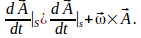## Transforming Velocities Between Rotating Coordinate Systems

Let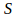and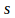be coordinate systems with the same origin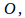with systemrotating with angular velocity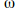with respect to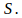Let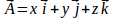be the position vector of a particle inthen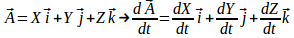In the coordinate system s,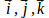are changing with time so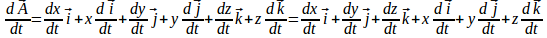Since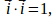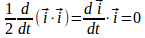so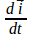and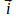are perpendicular. Similarly for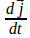and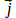and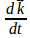and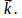Hence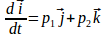(1)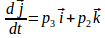(2)

,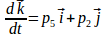(3)

Differentiating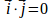gives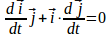(4)

Differentiating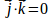gives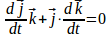(5)

Differentiating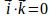gives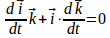(6)

Taking the dot product of (1) withgives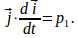Taking the dot product of (2) withgives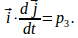Hence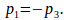Similarly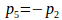and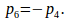Hence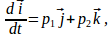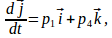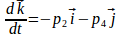Let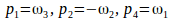then we can write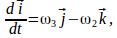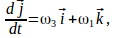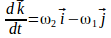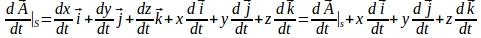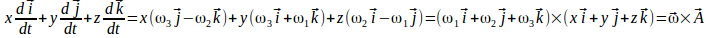Then